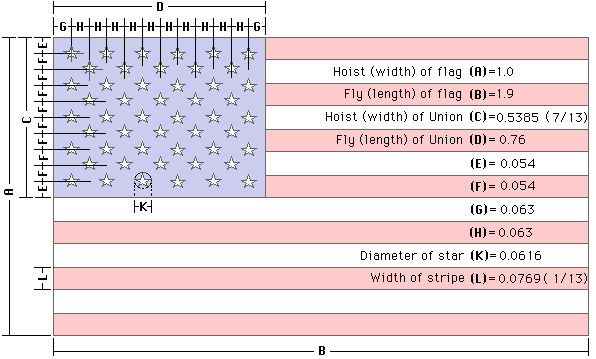Rate, Ratio, and Proportional Reasoning with Pop Art and Old Glory

Posted: October 19, 2015 in Advance, Off the Beaten Math, Periods 3&47.RP.A.1

Compute unit rates associated with ratios of fractions, including ratios of lengths, areas and other quantities measured in like or different units.

7.RP.A.2

Recognize and represent proportional relationships between quantities.

7.RP.A.2a

Decide whether two quantities are in a proportional relationship, e.g., by testing for equivalent ratios in a table or graphing on a coordinate plane and observing whether the graph is a straight line through the origin.

7.RP.A.2b

Identify the constant of proportionality (unit rate) in tables, graphs, equations, diagrams, and verbal descriptions of proportional relationships.

7.RP.A.2c

Represent proportional relationships by equations.

7.RP.A.2d

Explain what a point (x, y) on the graph of a proportional relationship means in terms of the situation, with special attention to the points (0, 0) and (1, r) where r is the unit rate.

7.RP.A.3

Use proportional relationships to solve multistep ratio and percent problems.